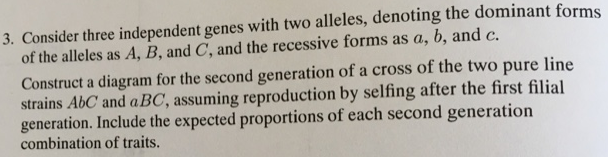# Problem: Consider three independent genes with two alleles, denoting the dominant forms of the alleles as A, B, and C, and the recessive forms as a, b, and c.Construct a diagram for the second generation of a cross of the two pure line strains AbC and aBC, assuming reproduction by selfing after the first filial generation. Include the expected proportions of each second generation combination of traits.

###### FREE Expert Solution

F0: AAbbCC x aaBBCC

F1: AaBbCC

For the second generation, the gametes will be ABC, AbC, aBC, and abC###### Problem Details

Consider three independent genes with two alleles, denoting the dominant forms of the alleles as A, B, and C, and the recessive forms as a, b, and c.

Construct a diagram for the second generation of a cross of the two pure line strains AbC and aBC, assuming reproduction by selfing after the first filial generation. Include the expected proportions of each second generation combination of traits.